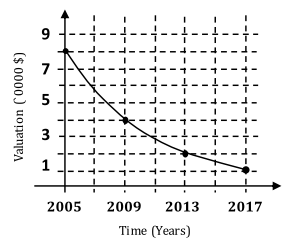# Free SAT Practice Questions

Question 1 of 1
ID: SAT-HOA-9
Section: Math - Heart of Algebra
Topics: Heart of Algebra; Graphic function
Difficulty level: Hard

(Practice Mode: Single selected Question » Back to Overview)The graph above shows the valuation of a car over the years. If $t$ represents the number of years starting from 2005, which of the following best describes the valuation of the car over the years? (without calculator)

A$\$\left(\dfrac{90000}{2^{\frac{4}{t}}}\right)$B$\$\left(\dfrac{80000}{2^{4t}}\right)$
C$\$\left(\dfrac{90000}{2^t}\right)$D$\$\left(\dfrac{80000}{2^{\frac{t}{4}}}\right)$
•